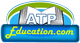ATP Education

Page 1 of 5

1. Chemical Reactions and Equations

Chapter Review

Chapter Review:

• Chemical changes are also known as chemical reaction.
• There are two parts of chemical reaction, (i) reactants (ii) products.
• The substances that undergo chemical change in the reaction are the reactants.
• The new substance, formed during the reaction, is the product.
• A word-equation shows change of reactants to products through an arrow placed between them.
• The arrowhead points towards the products, and shows the direction of the reaction.
• The reactants are written on the left-hand side (LHS) with a plus sign (+) between them. Similarly, products are written on the right-hand side (RHS) with a plus sign (+) between them.
• Chemical equations can be made more concise and useful if we use chemical formulae instead of words.
•  A chemical equation represents a chemical reaction.
• The number of atom of each element on both sides of arrow is equal.
• Unbalance chemical equation is called skeletal equation.
• Chemical equation is balanced to satisfy the law of conservation of mass.
• The total mass of the elements present in the products of a chemical reaction has to be equal to the total mass of the elements present in the reactants.
• The number of atoms of each element remains the same, before and after a chemical reaction.
• Skeletal equation can be balanced by using Hit and trial method or inspecting method.
• Combination reaction, Decomposition reaction, Displacement reaction, Double displacement reaction, oxidation and reduction reaction are the types of chemical reaction.
• Corrosion and rancidity are the effects of oxidation.
•  A complete chemical equation represents the reactants, products and their physical states symbolically.
• In a combination reaction two or more substances combine to form a new single substance.
• Reactions in which energy is absorbed are known as endothermic reactions.
• Precipitation reactions produce insoluble salts.
• Two different atoms or groups of atoms (ions) are exchanged in double displacement reactions.
• Reactions also involve the gain or loss of oxygen or hydrogen by substances. Oxidation is the gain of oxygen or loss of hydrogen. Reduction is the loss of oxygen or gain of hydrogen.
• When an element displaces another element from its compound, a displacement reaction occurs.

ATP Education
www.atpeducation.com ATP Education www.atpeducation.com

ATP Education

Page 1 of 5

See other sub-topics of this chapter: